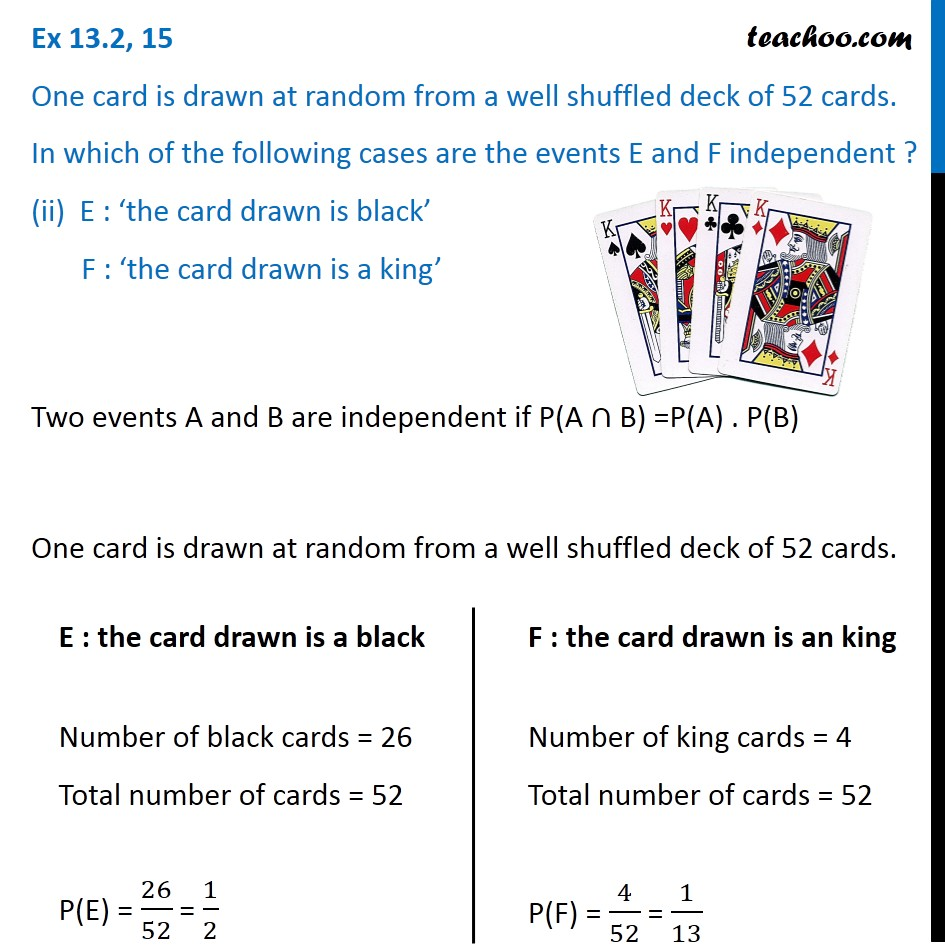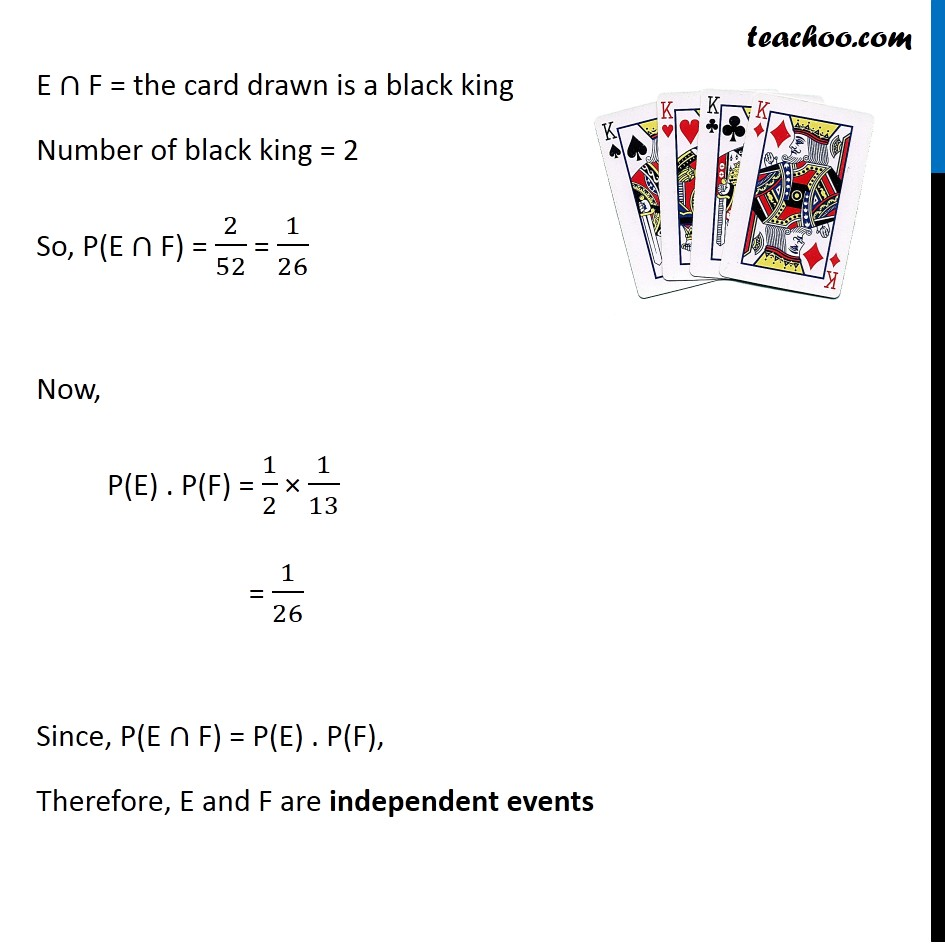Ex 13.2

Chapter 13 Class 12 Probability
Serial order wiseLearn in your speed, with individual attention - Teachoo Maths 1-on-1 Class

### Transcript

Ex 13.2, 15 One card is drawn at random from a well shuffled deck of 52 cards. In which of the following cases are the events E and F independent ? (ii) E : ‘the card drawn is black’ F : ‘the card drawn is a king’Finding P(E), P(F) and P(E ∩ F) E : the card drawn is a black Number of black cards = 26 Total number of cards = 52 P(E) = 26/52 = 1/2 F : the card drawn is an king Number of king cards = 4 Total number of cards = 52 P(F) = 4/52 = 1/13 Now, E ∩ F = the card drawn is a black king Number of black king = 2 So, P(E ∩ F) = 2/52 = 1/26 Now, P(E) . P(F) = 1/2 × 1/13 = 1/26 = P(E ∩ F) Since, P(E ∩ F) = P(E) . P(F), Therefore, E and F are independent events